# A closer look at orbit integration¶

## Orbit initialization¶

### Standard initialization¶

Orbits can be initialized in various coordinate frames. The simplest initialization gives the initial conditions directly in the Galactocentric cylindrical coordinate frame (or in the rectangular coordinate frame in one dimension). Orbit() automatically figures out the dimensionality of the space from the initial conditions in this case. In three dimensions initial conditions are given either as [R,vR,vT,z,vz,phi] or one can choose not to specify the azimuth of the orbit and initialize with [R,vR,vT,z,vz]. Since potentials in galpy are easily initialized to have a circular velocity of one at a radius equal to one, initial coordinates are best given as a fraction of the radius at which one specifies the circular velocity, and initial velocities are best expressed as fractions of this circular velocity. For example,

>>> from galpy.orbit import Orbit
>>> o= Orbit([1.,0.1,1.1,0.,0.1,0.])


initializes a fully three-dimensional orbit, while

>>> o= Orbit([1.,0.1,1.1,0.,0.1])


initializes an orbit in which the azimuth is not tracked, as might be useful for axisymmetric potentials.

In two dimensions, we can similarly specify fully two-dimensional orbits o=Orbit([R,vR,vT,phi]) or choose not to track the azimuth and initialize with o= Orbit([R,vR,vT]).

In one dimension we simply initialize with o= Orbit([x,vx]).

### Initialization with physical units¶

Orbits are normally used in galpy’s natural coordinates. When Orbits are initialized using a distance scale ro= and a velocity scale vo=, then many Orbit methods return quantities in physical coordinates. Specifically, physical distance and velocity scales are specified as

>>> op= Orbit([1.,0.1,1.1,0.,0.1,0.],ro=8.,vo=220.)


All output quantities will then be automatically be specified in physical units: kpc for positions, km/s for velocities, (km/s)^2 for energies and the Jacobi integral, km/s kpc for the angular momentum o.L() and actions, 1/Gyr for frequencies, and Gyr for times and periods. See below for examples of this.

The actual initial condition can also be specified in physical units. For example, the Orbit above can be initialized as

>>> from astropy import units
>>> op= Orbit([8.*units.kpc,22.*units.km/units.s,242*units.km/units.s,0.*units.kpc,22.*units.km/units.s,0.*units.deg])


In this case, it is unnecessary to specify the ro= and vo= scales; when they are not specified, ro and vo are set to the default values from the configuration file. However, if they are specified, then those values rather than the ones from the configuration file are used.

Tip

If you do input and output in physical units, the internal unit conversion specified by ro= and vo= does not matter!

Inputs to any Orbit method can also be specified with units as an astropy Quantity. galpy’s natural units are still used under the hood, as explained in the section on physical units in galpy. For example, integration times can be specified in Gyr if you want to integrate for a specific time period.

If for any output you do not want the output in physical units, you can specify this by supplying the keyword argument use_physical=False.

### Initialization from observed coordinates or astropy SkyCoord¶

For orbit integration and characterization of observed stars or clusters, initial conditions can also be specified directly as observed quantities when radec=True is set (see further down in this section on how to use an astropy SkyCoord instead). In this case a full three-dimensional orbit is initialized as o= Orbit([RA,Dec,distance,pmRA,pmDec,Vlos],radec=True) where RA and Dec are expressed in degrees, the distance is expressed in kpc, proper motions are expressed in mas/yr (pmra = pmra’ * cos[Dec] ), and Vlos is the heliocentric line-of-sight velocity given in km/s. The observed epoch is currently assumed to be J2000.00. These observed coordinates are translated to the Galactocentric cylindrical coordinate frame by assuming a Solar motion that can be specified as either solarmotion='hogg' (2005ApJ…629..268H), solarmotion='dehnen' (1998MNRAS.298..387D) or solarmotion='schoenrich' (default; 2010MNRAS.403.1829S). A circular velocity can be specified as vo=220 in km/s and a value for the distance between the Galactic center and the Sun can be given as ro=8.0 in kpc (e.g., 2012ApJ…759..131B). While the inputs are given in physical units, the orbit is initialized assuming a circular velocity of one at the distance of the Sun (that is, the orbit’s position and velocity is scaled to galpy’s natural units after converting to the Galactocentric coordinate frame, using the specified ro= and vo=). The parameters of the coordinate transformations are stored internally, such that they are automatically used for relevant outputs (for example, when the RA of an orbit is requested). An example of all of this is:

>>> o= Orbit([20.,30.,2.,-10.,20.,50.],radec=True,ro=8.,vo=220.)


However, the internally stored position/velocity vector is

>>> print(o.vxvv)
# [1.1480792664061401, 0.1994859759019009, 1.8306295160508093, -0.13064400474040533, 0.58167185623715167, 0.14066246212987227]


and is therefore in natural units.

Tip

Initialization using observed coordinates can also use units. So, for example, proper motions can be specified as 2*units.mas/units.yr.

Similarly, one can also initialize orbits from Galactic coordinates using o= Orbit([glon,glat,distance,pmll,pmbb,Vlos],lb=True), where glon and glat are Galactic longitude and latitude expressed in degrees, and the proper motions are again given in mas/yr ((pmll = pmll’ * cos[glat] ):

>>> o= Orbit([20.,30.,2.,-10.,20.,50.],lb=True,ro=8.,vo=220.)
>>> print(o.vxvv)
# [0.79959714332811838, 0.073287283885367677, 0.5286278286083651, 0.12748861331872263, 0.89074407199364924, 0.0927414387396788]


When radec=True or lb=True is set, velocities can also be specified in Galactic coordinates if UVW=True is set. The input is then [RA,Dec,distance,U,V,W], where the velocities are expressed in km/s. U is, as usual, defined as -vR (minus vR).

Finally, orbits can also be initialized using an astropy.coordinates.SkyCoord object. For example, the (ra,dec) example from above can also be initialized as:

>>> from astropy.coordinates import SkyCoord
>>> import astropy.units as u
>>> c= SkyCoord(ra=20.*u.deg,dec=30.*u.deg,distance=2.*u.kpc,
pm_ra_cosdec=-10.*u.mas/u.yr,pm_dec=20.*u.mas/u.yr,
>>> o= Orbit(c)


In this case, you can still specify the properties of the transformation to Galactocentric coordinates using the standard ro, vo, zo, and solarmotion keywords, or you can use the SkyCoord Galactocentric frame specification and these are propagated to the Orbit instance. For example,

>>> from astropy.coordinates import CartesianDifferential
>>> c= SkyCoord(ra=20.*u.deg,dec=30.*u.deg,distance=2.*u.kpc,
pm_ra_cosdec=-10.*u.mas/u.yr,pm_dec=20.*u.mas/u.yr,
galcen_distance=8.*u.kpc,z_sun=15.*u.pc,
galcen_v_sun=CartesianDifferential([10.0,235.,7.]*u.km/u.s))
>>> o= Orbit(c)


A subtlety here is that the galcen_distance and ro keywords are not interchangeable, because the former is the distance between the Sun and the Galactic center and ro is the projection of this distance onto the Galactic midplane. Another subtlety is that the astropy Galactocentric frame is a right-handed frame, while galpy normally uses a left-handed frame, so the sign of the x component of galcen_v_sun is the opposite of what it would be in solarmotion. Because the Galactocentric frame in astropy does not specify the circular velocity, but only the Sun’s velocity, you still need to specify vo to use a non-default circular velocity.

When orbits are initialized using radec=True, lb=True, or using a SkyCoord physical scales ro= and vo= are automatically specified (because they have defaults of ro=8 and vo=220). Therefore, all output quantities will be specified in physical units (see above). If you do want to get outputs in galpy’s natural coordinates, you can turn this behavior off by doing

>>> o.turn_physical_off()


All outputs will then be specified in galpy’s natural coordinates.

### Initializing multiple objects at once¶

In all of the examples above, the Orbit instance corresponds to a single object, but Orbit instances can also contain and analyze multiple objects at once. This makes handling Orbit instances highly convenient and also allows for efficient handling of multiple objects. Many of the most computationally-intense methods have been parallelized (orbit integration; analytic eccentricity, zmax, etc. calculation; action-angle calculations) and some other methods switch to more efficient algorithms for larger numbers of objects (e.g., rguiding, rE, LcE).

All of the methods for initializing Orbit instances above work for multiple objects. Specifically, the initial conditions can be:

• Array of arbitrary shape (shape,phasedim); needs to be in internal units (for Quantity input; see ‘list’ option below or use a SkyCoord):
• in Galactocentric cylindrical coordinates with phase-space coordinates arranged as [R,vR,vT(,z,vz,phi)];

• [ra,dec,d,mu_ra, mu_dec,vlos] or [l,b,d,mu_l, mu_b, vlos] in [deg,deg,kpc,mas/yr,mas/yr,km/s], or [ra,dec,d,U,V,W] or [l,b,d,U,V,W] in [deg,deg,kpc,km/s,km/s,kms] (ICRS where relevant; mu_ra = mu_ra * cos dec and mu_l = mu_l * cos ); use the radec=, lb=, and UVW= keywords as before

• astropy (>v3.0) SkyCoord with arbitrary shape, including velocities;

• lists of initial conditions, entries can be
• individual Orbit instances (of single objects)

• Regular or Quantity arrays arranged as in the first bullet above (so things like [R,vR,vT,z,vz,phi], where R, vR, … can be arbitrary shape regular or Quantity arrays)

• list of Quantities (so things like [R1,vR1,..,], where R1, vR1, … are scalar Quantities

• None: assumed to be the Sun; if None occurs in a list it is assumed to be the Sun and all other items in the list are assumed to be [ra,dec,…]; cannot be combined with Quantity lists

• lists of scalar phase-space coordinates arranged as in the first bullet above (so things like [R,vR,…] where R,vR are scalars in internal units

Tip

For multiple object initialization using an array or SkyCoord, arbitrary input shapes are supported.

An example initialization with an array is:

>>> vxvvs= numpy.array([[1.,0.1,1.,0.1,-0.2,1.5],[0.1,0.3,1.1,-0.3,0.4,2.]])
>>> orbits= Orbit(vxvvs)
>>> print(orbits.R())
# [ 1.   0.1]


and with a SkyCoord:

>>> numpy.random.seed(1)
>>> nrand= 30
>>> ras= numpy.random.uniform(size=nrand)*360.*u.deg
>>> decs= 90.*(2.*numpy.random.uniform(size=nrand)-1.)*u.deg
>>> dists= numpy.random.uniform(size=nrand)*10.*u.kpc
>>> pmras= 20.*(2.*numpy.random.uniform(size=nrand)-1.)*20.*u.mas/u.yr
>>> pmdecs= 20.*(2.*numpy.random.uniform(size=nrand)-1.)*20.*u.mas/u.yr
>>> vloss= 200.*(2.*numpy.random.uniform(size=nrand)-1.)*u.km/u.s
# Without any custom coordinate-transformation parameters
>>> co= SkyCoord(ra=ras,dec=decs,distance=dists,
pm_ra_cosdec=pmras,pm_dec=pmdecs,
frame='icrs')
>>> orbits= Orbit(co)
>>> print(orbits.ra()[:3],ras[:3])
# [  1.50127922e+02   2.59316818e+02   4.11749371e-02] deg [  1.50127922e+02   2.59316818e+02   4.11749342e-02] deg


As before, you can use the SkyCoord Galactocentric frame specification here.

Orbit instances containing multiple objects act like numpy arrays in many ways, but have some subtly different behaviors for some functions. For example, one can do:

>>> print(len(orbits))
# 30
>>> print(orbits.shape)
# (30,)
>>> print(orbits.size)
# 30
>>> orbits.reshape((6,5)) # reshape is done inplace
>>> print(len(orbits))
# 6
>>> print(orbits.shape)
# (6,5)
>>> print(orbits.size)
# 30
>>> sliced_orbits= orbits[:3,1:5] # Extract a subset using numpy's slicing rules
>>> print(sliced_orbits.shape)
# (3,4)
>>> single_orbit= orbits[1,3] # Extract a single object
>>> print(single_orbit.shape)
# ()


Slicing creates a new Orbit instance. When slicing an Orbit instance that has been integrated, the integrated orbit will be transferred to the new instance.

The shape of the Orbit instances is retained for all relevant outputs. Continuing on from the previous example (where orbits has shape (6,5) after we reshaped it), we have:

>>> print(orbits.R().shape)
# (6,5)
>>> print(orbits.L().shape)
# (6,5,3)


After orbit integration, evaluating orbits.R(times) would return an array with shape (6,5,ntimes) here.

### Initialization from an object’s name¶

A convenience method, Orbit.from_name, is also available to initialize orbits from the name of an object. For example, for the star Lacaille 8760:

>>> o= Orbit.from_name('Lacaille 8760', ro=8., vo=220.)
>>> [o.ra(), o.dec(), o.dist(), o.pmra(), o.pmdec(), o.vlos()]
# [319.31362023999276, -38.86736390000036, 0.003970940656277758, -3258.5529999996584, -1145.3959999996205, 20.560000000006063]


but this also works for globular clusters, e.g., to obtain Omega Cen’s orbit and current location in the Milky Way do:

>>> o= Orbit.from_name('Omega Cen')
>>> from galpy.potential import MWPotential2014
>>> ts= numpy.linspace(0.,100.,2001)
>>> o.integrate(ts,MWPotential2014)
>>> o.plot()
>>> plot([o.R()],[o.z()],'ro')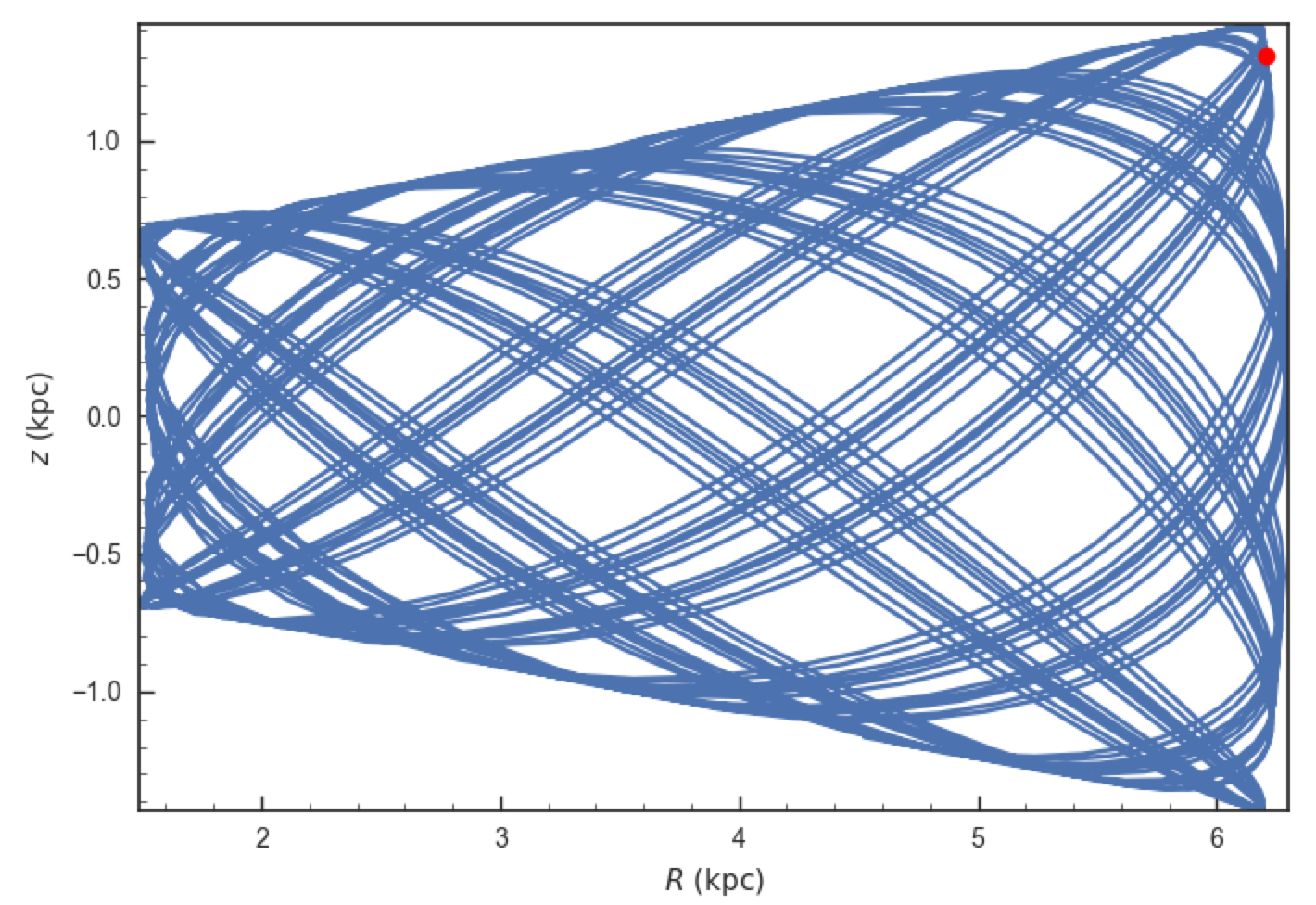We see that Omega Cen is currently close to its maximum distance from both the Galactic center and from the Galactic midplane (note that Omega Cen’s phase-space coordinates were updated internally in galpy after this plot was made and the orbit is now slightly different).

Similarly, you can do:

>>> o= Orbit.from_name('LMC')
>>> [o.ra(), o.dec(), o.dist(), o.pmra(), o.pmdec(), o.vlos()]
# [80.894200000000055, -69.756099999999847, 49.999999999999993, 1.909999999999999, 0.2290000000000037, 262.19999999999993]


It is also possible to initialize using multiple names, for example:

>>> o= Orbit.from_name(['LMC','SMC'])
>>> print(o.ra(),o.dec(),o.dist())
# [ 80.8942  13.1583] deg [-69.7561 -72.8003] deg [ 50.  60.] kpc


The names are stored in the name attribute:

>>> print(o.name)
# ['LMC', 'SMC']


The Orbit.from_name method attempts to resolve the name of the object in SIMBAD, and then use the observed coordinates found there to generate an Orbit instance. In order to query SIMBAD, Orbit.from_name requires the astroquery package to be installed. A small number of objects, mainly Milky Way globular clusters and dwarf satellite galaxies, have their phase-space coordinates stored in a file that is part of galpy and for these objects the values from this file are used rather than querying SIMBAD. Orbit.from_name supports tab completion in IPython/Jupyter for this list of objects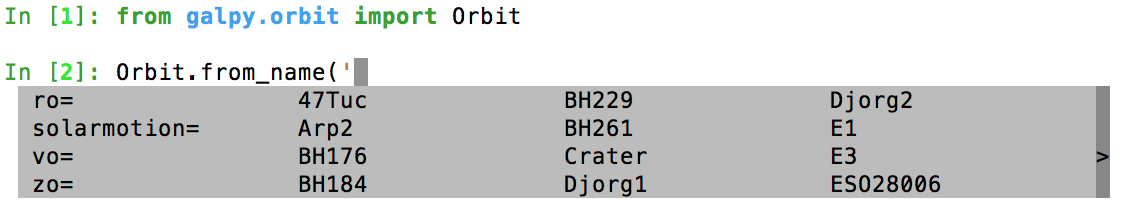The Orbit.from_name method also allows you to load some collections of objects in a simple manner. Currently, three collections are supported: ‘MW globular clusters’, ‘MW satellite galaxies’, and ‘solar system’. Specifying ‘MW globular clusters’ loads all of the Milky-Way globular clusters with data from Gaia EDR3:

>>> o= Orbit.from_name('MW globular clusters')
>>> print(len(o))
# 161
>>> print(o.name)
# ['NGC5286' 'Terzan12' 'Arp2', ... ]
>>> print(o.r())
# [  8.4418065    2.99042499  21.55042257 ...]


It is then easy to, for example, integrate the orbits of all Milky-Way globular clusters in MWPotential2014 and plot them in 3D:

>>> ts= numpy.linspace(0.,300.,1001)
>>> o.integrate(ts,MWPotential2014)
>>> o.plot3d(alpha=0.4)
>>> xlim(-100.,100.)
>>> ylim(-100.,100)
>>> gca().set_zlim3d(-100.,100);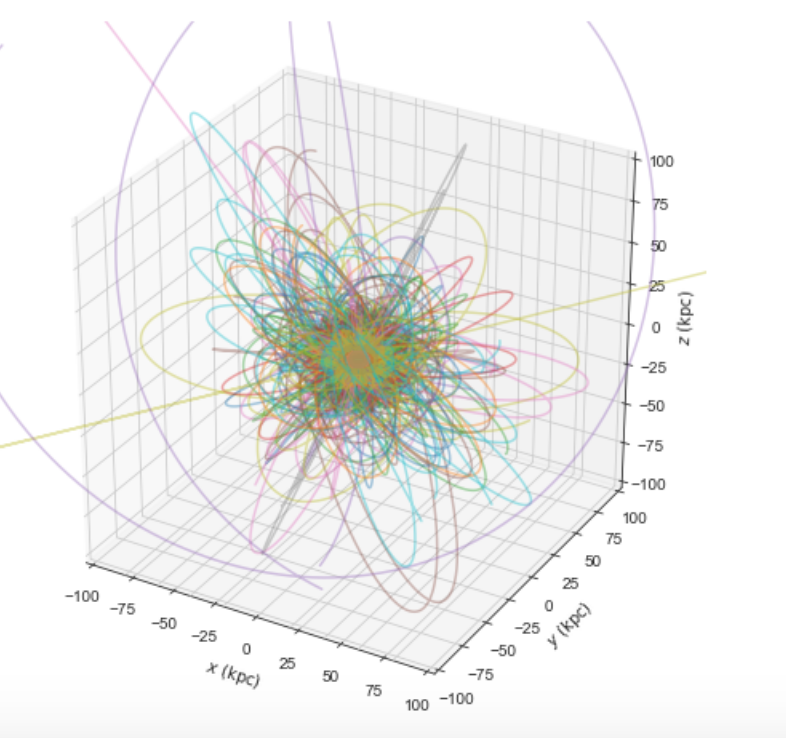Similarly, ‘MW satellite galaxies’ loads all of the Milky-Way satellite galaxies from Pace et al. (2022):

>>> o= Orbit.from_name('MW satellite galaxies')
>>> print(len(o))
# 50
>>> print(o.name)
# ['AntliaII' 'AquariusII' 'BootesI' 'BootesII' 'BootesIII' 'CanesVenaticiI'
'CanesVenaticiII' 'Carina' 'CarinaII' 'CarinaIII' 'ColumbaI'
'ComaBerenices' 'CraterII' 'Draco' 'DracoII' 'EridanusII' 'Fornax'
'GrusI' 'GrusII' 'Hercules' 'HorologiumI' 'HydraII' 'HydrusI' 'LMC'
'LeoI' 'LeoII' 'LeoIV' 'LeoV' 'PegasusIII' 'PhoenixI' 'PhoenixII'
'PiscesII' 'ReticulumII' 'ReticulumIII' 'SMC' 'SagittariusII' 'Sculptor'
'Segue1' 'Segue2' 'Sextans' 'Sgr' 'TriangulumII' 'TucanaII' 'TucanaIII'
'TucanaIV' 'TucanaV' 'UrsaMajorI' 'UrsaMajorII' 'UrsaMinor' 'Willman1']
>>> print(o.r())
# [132.93721433 105.41442453  63.66115037  39.83901891  45.52928256
209.7700823  160.60534628 107.16399152  38.24845108  28.9274277
187.46809402  43.16546984 116.44003784  75.80593376  23.71949352
367.79884477 149.18728196 123.27421442  50.52704455 125.11664692
79.292941   148.20468685  25.74545627  49.60813235 261.93577755
235.49080095 151.95997497 169.77910104 213.08771512 418.76813979
81.18196715 182.15380106  32.72475876  91.96956471  60.28760354
63.35088903  83.99141137  27.77404178  42.42833236  95.44075955
19.06561359  34.54212398  54.28214129  21.05199179  44.4906331
51.92586773 101.87656784  40.73034335  78.06428801  42.6385771 ] kpc


and we can integrate and plot them in 3D as above:

>>> o.plot3d(alpha=0.4)
>>> xlim(-400.,400.)
>>> ylim(-400.,400)
>>> gca().set_zlim3d(-400.,400)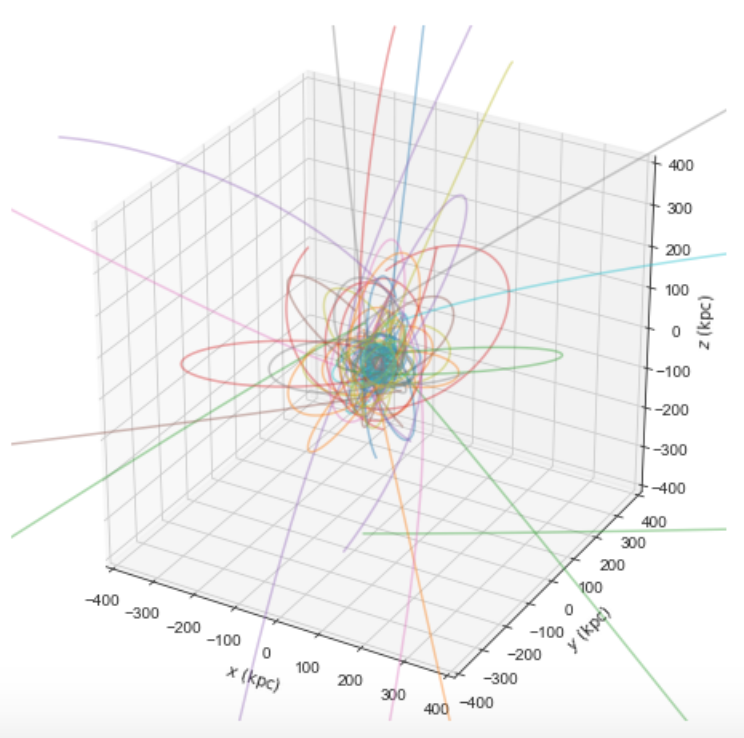Because MWPotential2014 has a relatively low-mass dark-matter halo, a bunch of the satellites are unbound (to make them bound, you can increase the mass of the halo by, for example, multiplying it by 1.5, as in MWPotential2014*= 1.5).

Finally, for illustrative purposes, the solar system is included as a collection as well. The solar system is set up such that the center of what is normally the Galactocentric coordinate frame in galpy is now the solar system barycenter and the coordinate frame is a heliocentric one. The solar system data are taken from Bovy et al. (2010) and they represent the positions and planets on April 1, 2009. To load the solar system do:

>>> o= Orbit.from_name('solar system')


Giving for example:

>>> print(o.name)
# ['Mercury', 'Venus', 'Earth', 'Mars', 'Jupiter', 'Saturn', 'Uranus', 'Neptune']


You can then, for example, integrate the solar system for 10 years as follows

>>> import astropy.units as u
>>> from galpy.potential import KeplerPotential
>>> from galpy.util.conversion import get_physical
>>> kp= KeplerPotential(amp=1.*u.Msun,**get_physical(o)) # Need to use **get_physical to get the ro= and vo= parameters, which differ from the default for the solar system
>>> ts= numpy.linspace(0.,10.,1001)*u.yr
>>> o.integrate(ts,kp)
>>> o.plot(d1='x',d2='y')


which gives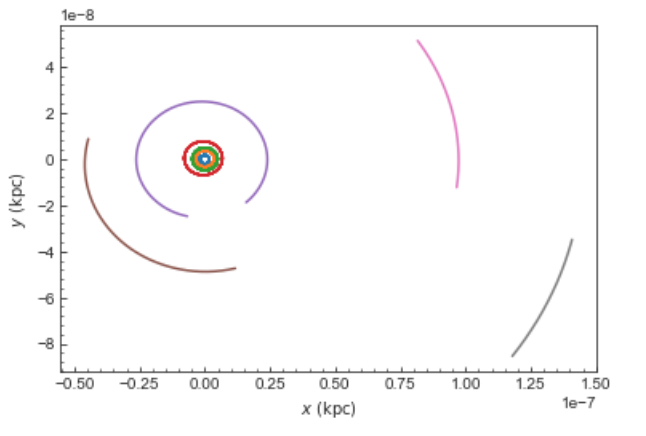Note that, as usual, physical outputs are in kpc, leading to very small numbers!

Tip

Setting up an Orbit instance without arguments will return an Orbit instance representing the Sun: o= Orbit(). This instance has physical units turned on by default, so methods will return outputs in physical units unless you o.turn_physical_off().

Warning

Orbits initialized using Orbit.from_name have physical output turned on by default, so methods will return outputs in physical units unless you o.turn_physical_off().

## Orbit integration¶

After an orbit is initialized, we can integrate it for a set of times ts, given as a numpy array. For example, in a simple logarithmic potential we can do the following

>>> from galpy.potential import LogarithmicHaloPotential
>>> lp= LogarithmicHaloPotential(normalize=1.)
>>> o= Orbit([1.,0.1,1.1,0.,0.1,0.])
>>> import numpy
>>> ts= numpy.linspace(0,100,10000)
>>> o.integrate(ts,lp)


to integrate the orbit from t=0 to t=100, saving the orbit at 10000 instances. In physical units, we can integrate for 10 Gyr as follows

>>> from astropy import units
>>> ts= numpy.linspace(0,10.,10000)*units.Gyr
>>> o.integrate(ts,lp)


Warning

When the integration times are not specified using a Quantity, they are assumed to be in natural units.

If we initialize the Orbit using a distance scale ro= and a velocity scale vo=, then Orbit plots and outputs will use physical coordinates (currently, times, positions, and velocities)

>>> op= Orbit([1.,0.1,1.1,0.,0.1,0.],ro=8.,vo=220.) #Use Vc=220 km/s at R= 8 kpc as the normalization
>>> op.integrate(ts,lp)


An Orbit instance containing multiple objects can be integrated in the same way and the orbit integration will be performed in parallel on machines with multiple cores. For the fast C integrators (see below), this parallelization is done using OpenMP in C and requires one to set the OMP_NUM_THREADS environment variable to control the number of cores used. The Python integrators are parallelized in Python and by default also use the OMP_NUM_THREADS variable to set the number of cores (but for the Python integrators this can be overwritten). A simple example is

>>> vxvvs= numpy.array([[1.,0.1,1.,0.1,-0.2,1.5],[0.1,0.3,1.1,-0.3,0.4,2.]])
>>> orbits= Orbit(vxvvs)
>>> orbits.integrate(ts,lp)
>>> print(orbits.R(ts).shape)
# (2,10000)
>>> print(orbits.R(ts))
# [[ 1.          1.00281576  1.00563403 ...,  1.05694767  1.05608923
#   1.0551804 ]
# [ 0.1         0.18647825  0.27361065 ...,  3.39447863  3.34992543
#   3.30527001]]


## Orbit integration in non-inertial frames¶

The default assumption in galpy is that the frame that an orbit is integrated in is an inertial one. However, galpy also supports orbit integration in non-inertial frames that are rotating or whose center is accelerating (or a combination of the two). When a frame is not an inertial frame, fictitious forces such as the centrifugal and Coriolis forces need to be taken into account. galpy implements all of the necessary forces as part of the NonInertialFrameForce class. objects of this class are instantiated with arbitrary three-dimensional rotation frequencies (and their time derivative) and/or arbitrary three-dimensional acceleration of the origin. The class documentation linked to above provides full mathematical details on the rotation and acceleration of the non-inertial frame.

We can then, for example, integrate the orbit of the Sun in the LSR frame, that is, the frame that is corotating with that of the circular orbit at the location of the Sun. To do this for MWPotential2014, do

>>> from galpy.potential import MWPotential2014, NonInertialFrameForce
>>> nip= NonInertialFrameForce(Omega=1.) # LSR has Omega=1 in natural units
>>> o= Orbit() # Orbit() is the orbit of the Sun in the inertial frame
>>> o.turn_physical_off() # To use internal units
>>> o= Orbit([o.R(),o.vR(),o.vT()-1.,o.z(),o.vz(),o.phi()]) # Convert to the LSR frame
>>> ts= numpy.linspace(0.,20.,1001)
>>> o.integrate(ts,MWPotential2014+nip)
>>> o.plot(d1='x',d2='y')


which gives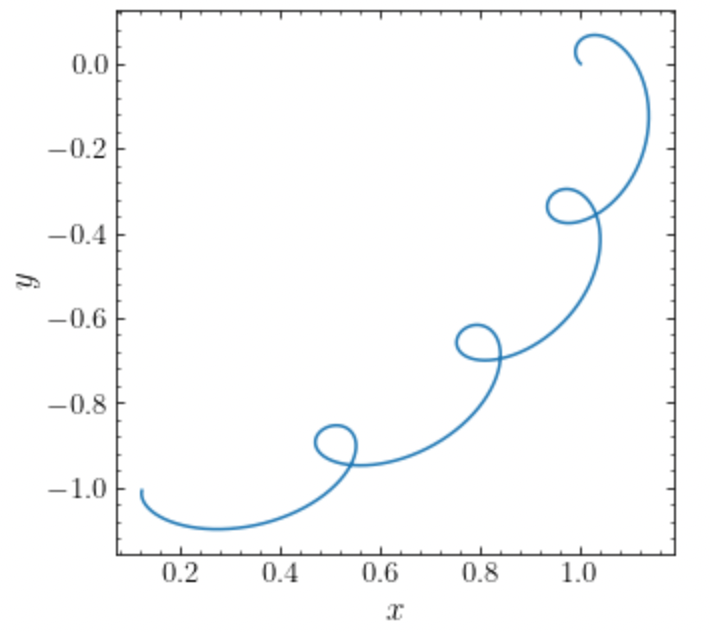we can compare this to integrating the orbit in the inertial frame and displaying it in the non-inertial LSR frame as follows:

>>> o.plot(d1='x',d2='y') # Repeat plot from above
>>> o= Orbit() # Orbit() is the orbit of the Sun in the inertial frame
>>> o.turn_physical_off() # To use internal units
>>> o.integrate(ts,MWPotential2014)
>>> o.plot(d1='R*cos(phi-t)',d2='R*sin(phi-t)',overplot=True) # Omega = 1, so Omega t = t


which gives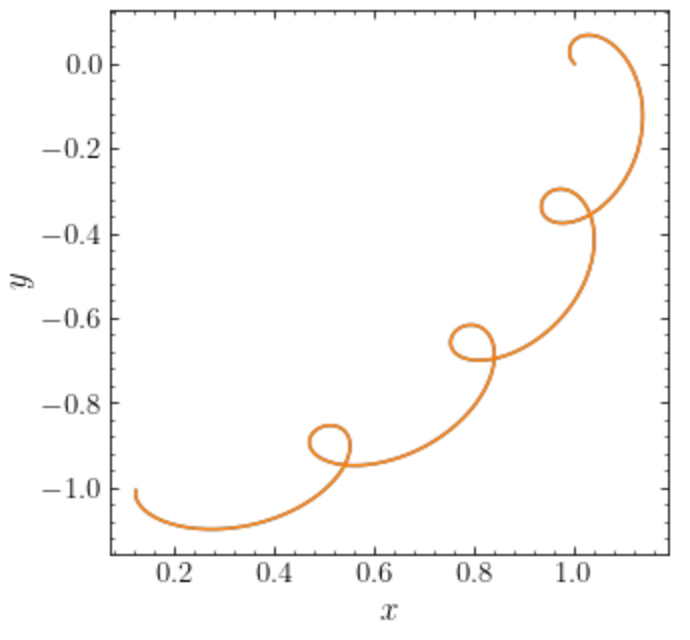We can also do all of the above in physical units, in which case the first example above becomes

>>> from galpy.potential import MWPotential2014, NonInertialFrameForce
>>> from astropy import units
>>> nip= NonInertialFrameForce(Omega=220./8.*units.km/units.s/units.kpc)
>>> o= Orbit() # Orbit() is the orbit of the Sun in the inertial frame
>>> o= Orbit([o.R(quantity=True),o.vR(quantity=True),
o.vT(quantity=True)-220.*units.km/units.s,
o.z(quantity=True),o.vz(quantity=True),
o.phi(quantity=True)]) # Convert to the LSR frame
>>> ts= numpy.linspace(0.,20.,1001)
>>> o.integrate(ts,MWPotential2014+nip)
>>> o.plot(d1='x',d2='y')


We can also provide the Omega= frequency as an arbitrary function of time. In this case, the frequency must be returned in internal units and the input time of this function must be in internal units as well (use the routines in galpy.util.conversion for converting from physical to internal units; you need to divide by these to go from physical to internal). For the example above, this would amount to setting

>>> nip= NonInertialFrameForce(Omega=lambda t: 1.,Omegadot=lambda t: 0.)


Note that when we supply Omega as a function, it is necessary to specify its time derivative as well as Omegadot (all again in internal units).

We give an example of having the origin of the non-inertial frame accelerate in the Example: Including the Milky Way center’s barycentric acceleration due to the Large Magellanic Cloud in orbit integrations section below.

## Displaying the orbit¶

After integrating the orbit, it can be displayed by using the plot() function. The quantities that are plotted when plot() is called depend on the dimensionality of the orbit: in 3D the (R,z) projection of the orbit is shown; in 2D either (X,Y) is plotted if the azimuth is tracked and (R,vR) is shown otherwise; in 1D (x,vx) is shown. E.g., for the example given above at the start of the Orbit integration section above,

>>> o.plot()


gives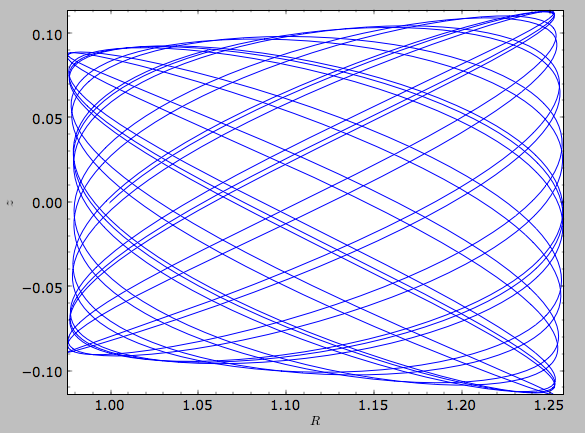If we do the same for the Orbit that has physical distance and velocity scales associated with it, we get the following

>>> op.plot()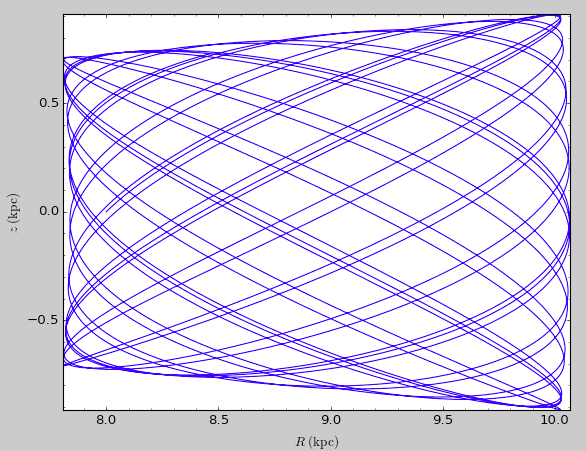If we call op.plot(use_physical=False), the quantities will be displayed in natural galpy coordinates.

Plotting an Orbit instance that consists of multiple objects plots all objects at once, e.g.,

>>> orbits.plot()


gives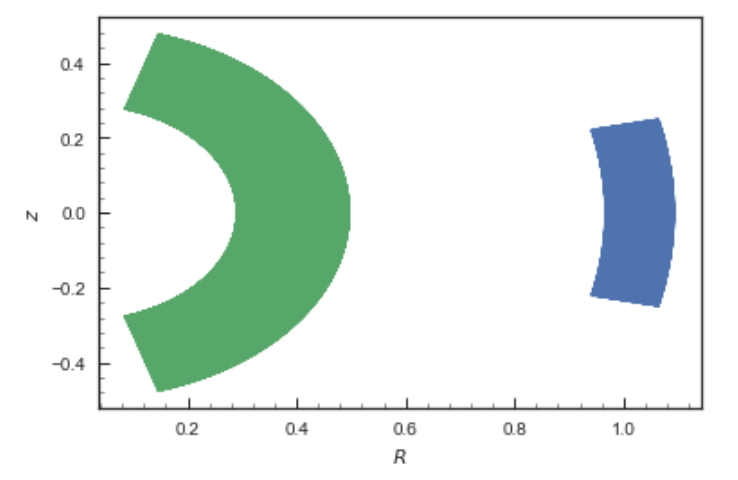Other projections of the orbit can be displayed by specifying the quantities to plot. E.g.,

>>> o.plot(d1='x',d2='y')


gives the projection onto the plane of the orbit: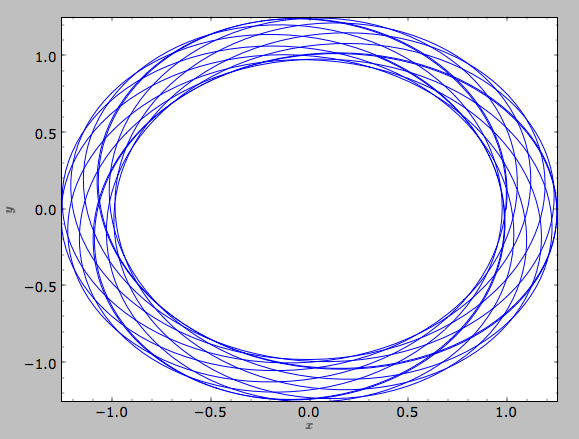while

>>> o.plot(d1='R',d2='vR')


gives the projection onto (R,vR):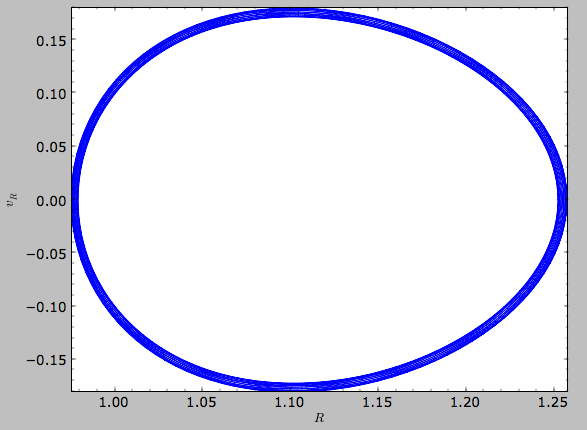We can also plot the orbit in other coordinate systems such as Galactic longitude and latitude

>>> o.plot('k.',d1='ll',d2='bb')


which shows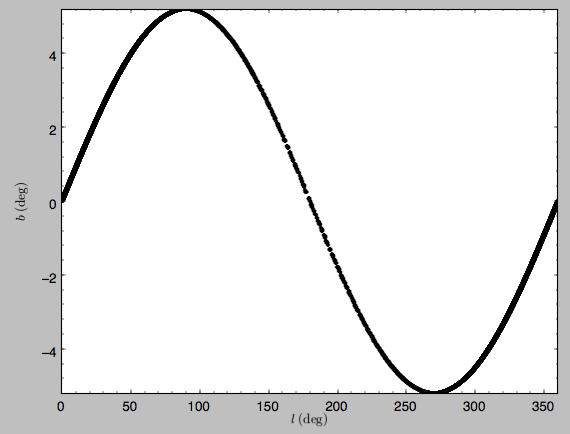or RA and Dec

>>> o.plot('k.',d1='ra',d2='dec')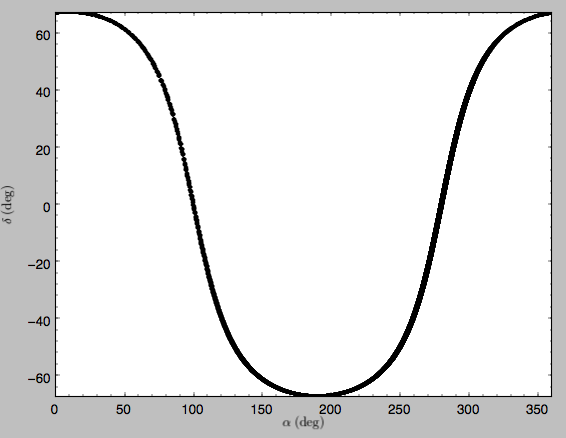See the documentation of the o.plot function and the o.ra(), o.ll(), etc. functions on how to provide the necessary parameters for the coordinate transformations.

It is also possible to plot quantities computed from the basic Orbit outputs like o.x(), o.r(), etc. For this to work, the numexpr module needs to be installed; this can be done using pip or conda. Then you can ask for plots like

>>> o.plot(d1='r',d2='vR*R/r+vz*z/r')


where d2= converts the velocity to spherical coordinates (this is currently not a pre-defined option). This gives the following orbit (which is closed in this projection, because we are using a spherical potential):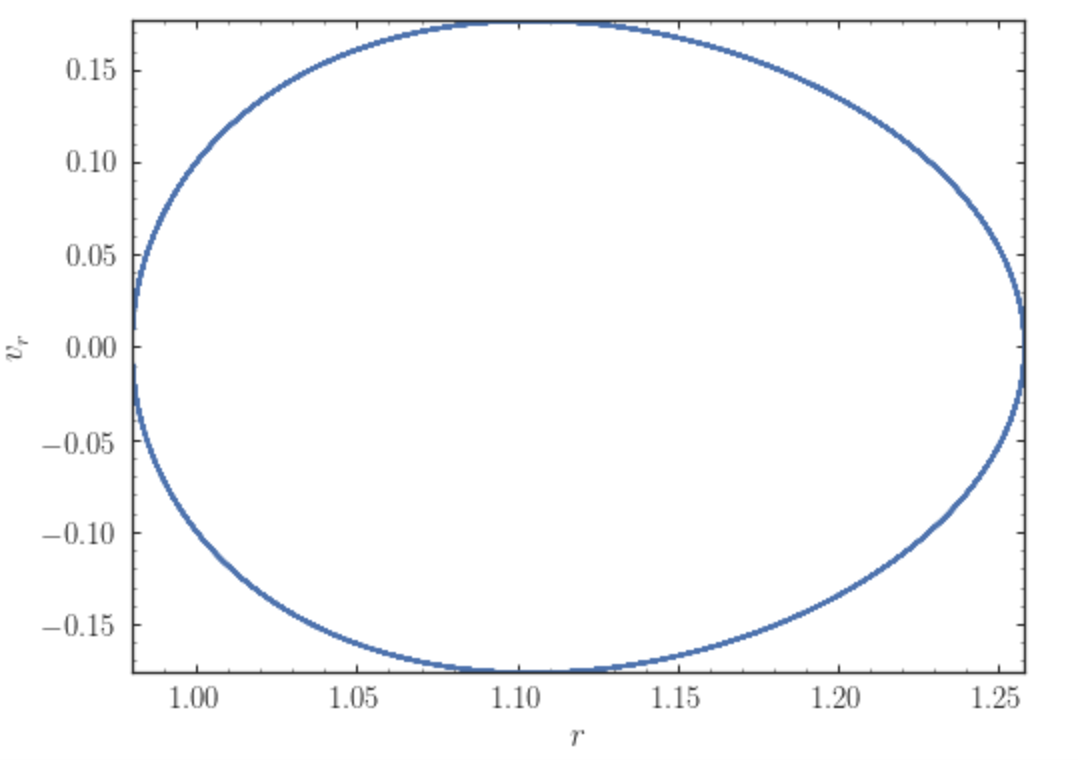You can also do more complex things like

>>> o.plot(d1='x',d2='y')
>>> o.plot(d1='R*cos(phi-{:f}*t)'.format(o.Op(quantity=False)),
d2='R*sin(phi-{:f}*t)'.format(o.Op(quantity=False)),
overplot=True)


which shows the orbit in the regular (x,y) frame as well as in a (x,y) frame that is rotating at the angular frequency of the orbit. When doing more complex calculations like this, you need to make sure that you are getting the units right: parameters param in the expression you provide are directly evaluated as o.param(), which depending on how you setup the object may or may not return output in physical units. The expression above is safe, because o.Op evaluated like this will be in a consistent unit system with the rest of the expression. Expressions cannot contain astropy Quantities (these cannot be parsed by the parser), which is why quantity=False is specified; this is also used internally.

Finally, it is also possible to plot arbitrary functions of time with Orbit.plot, by specifying d1= or d2= as a function. For example, to display the orbital velocity in the spherical radial direction, which we also did with the expression above, you can do the following

>>> o.plot(d1='r',
d2=lambda t: o.vR(t)*o.R(t)/o.r(t)+o.vz(t)*o.z(t)/o.r(t),
ylabel='v_r')


For a function like this, just specifying it as the expression d2='vR*R/r+vz*z/r' is much more convenient, but expressions that cannot be parsed automatically could be directly given as a function.

## Animating the orbit¶

Warning

Animating orbits is a new, experimental feature at this time that may be changed in later versions. It has only been tested in a limited fashion. If you are having problems with it, please open an Issue and list all relevant details about your setup (python version, jupyter version, browser, any error message in full). It may also be helpful to check the javascript console for any errors.

In a jupyter notebook or in jupyterlab (jupyterlab versions >= 0.33) you can also create an animation of an orbit after you have integrated it. For example, to do this for the op orbit from above (but only integrated for 2 Gyr to create a shorter animation as an example here), do

>>> op.animate()


This will create the following animation

Tip

There is currently no option to save the animation within galpy, but you could use screen capture software (for example, QuickTime’s Screen Recording feature) to record your screen while the animation is running and save it as a video.

animate has options to specify the width and height of the resulting animation, and it can also animate up to three projections of an orbit at the same time. For example, we can look at the orbit in both (x,y) and (R,z) at the same time with

>>> op.animate(d1=['x','R'],d2=['y','z'],width=800)


which gives

You can also animate orbit in 3D with an optional Milky Way galaxy centered at the origin

>>> op.animate3d(mw_plane_bg=True)


which gives